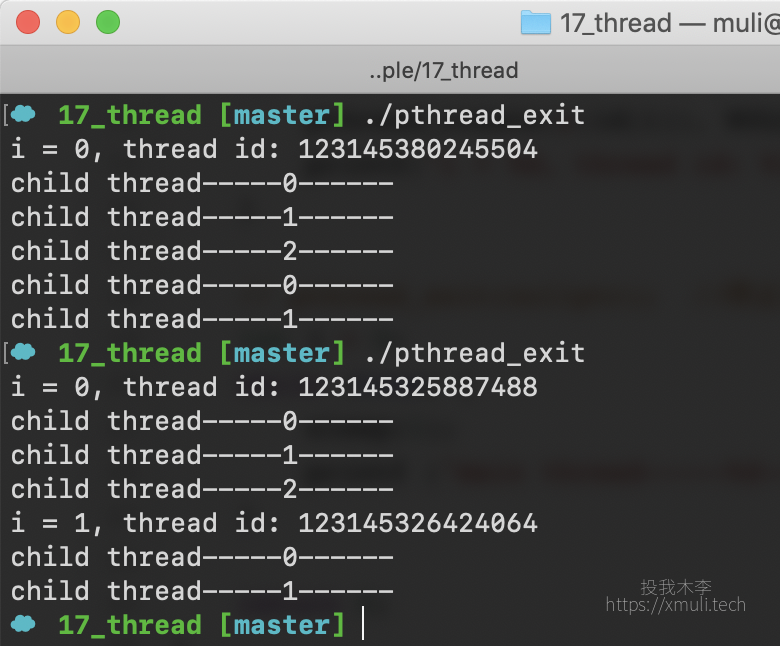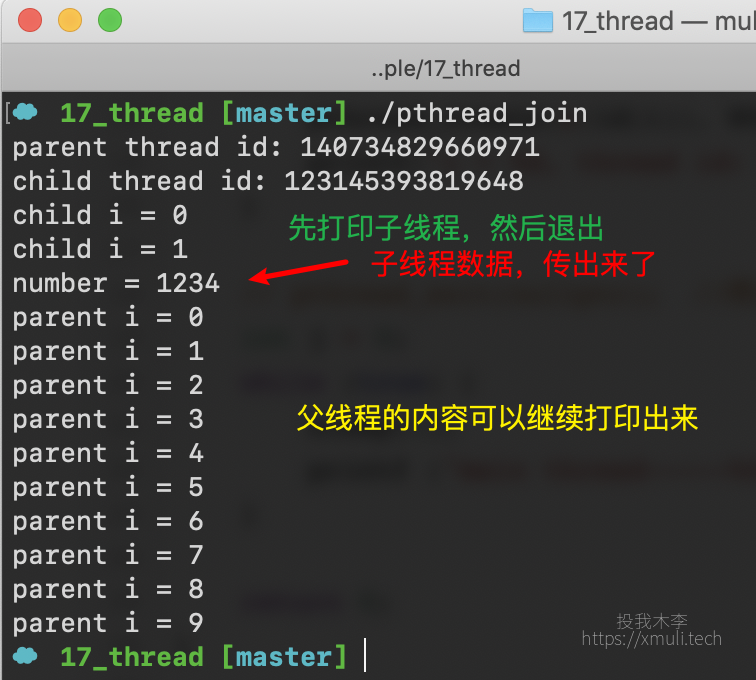简 述：　上一篇讲解了多线程的入门的知识，如何创建多线程程序，以及从虚拟地址空间和 PCB 分析线程的一些细节。本章再讲述一些线程操作相关的函数：

[TOC]

### 编程环境：

💻： `uos20` 📎 `gcc/g++ 8.3` 📎 `gdb8.0`

💻： `MacOS 10.14 ` 📎 `gcc/g++ 9.2` 📎 `gdb8.3`

• 作用： 一个进程的退出使用 `exit(0)`； 而退出一个线程，使用 `pthread_exit(NULL)`
``void pthread_exit(void *value_ptr);``
• 参数：

• value_ptr：必须指向全局，或者堆。是一个传出参数，用来在该线程结束推出的时候，传输一个内容。若是指向局部变量，该线程被销毁了的话，其他线程可能访问不到此块数据块。
• 写一个例子：

``````#include <stdio.h>
#include <unistd.h>

int num = 13; //设置为全局变量，在全局区域，共享

void* myfun(void* arg);

int main(int argc, char *argv[])
{
void* p = (void *)&num;  //传一个地址进去（voi* 也是 4 个字节）
for (int i = 0; i < 5; i++) {
printf("i = %d, thread id: %ld\n", i, id[i]);
}

int j = 0;
while (true) {
sleep(1);
}

return 0;
}

void* myfun(void* arg)
{
for (int i = 0; i < 5; i++) {

if (i == 2) {
_exit(0); //退出进程
}
}

return nullptr;
}``````
• 运行结果：• 作用:

阻塞等待线程退出，获取线程退出的状态

``int pthread_join(pthread_t thread, void **value_ptr);``
• 参数：

• 写了一例子：

``````#include <stdio.h>
#include <string.h>
#include <unistd.h>

int number = 1234; //要是一个全局的变量哦，或者是堆的空间
void* myfun(void* arg);

int main(int argc, char *argv[])
{

if (ret != 0)
printf("error: %s\n", strerror(ret));

void* ptr = nullptr;

printf("number = %d\n", *((int*)ptr));

int i = 0;
while (i < 10 ) {
printf("parent i = %d\n", i++);
}

return 0;
}

void* myfun(void* arg)
{

for (int i = 0; i < 5; i++) {
if (i == 2) {
// int number = 1234;   //若是为栈里面的数据，则会组线程里面，会崩溃或者失败
}

printf("child i = %d\n", i);
}

return NULL;
}``````
• 运行效果：``int pthread_detach(pthread_t thread);``

``int pthread_cancel(pthread_t thread);``
• 注意：
• 要杀死子线程对应的处理的函数的内部，必须做一次系统调用。
• 系统调用函数有，eg: open,write, printf 等，这次都是最终会调用到系统层及的函数。
• 不涉及到系统层级调用，eg： int a = 10;

``int pthread_equal(pthread_t t1, pthread_t t2);``